Molecular Structures Using VSEPR Lab Report

Molecular Structures Using VSEPR

https://mediaspace.minnstate.edu/media/1_h8xco6hz

In this lab activity, you will determine the Lewis structures and the three-dimensional geometries of molecular species given only their molecular formulas. This will help you perfect your skill at drawing Lewis structures and help you become better acquainted with molecular geometry by constructing models of the various geometries. You may work in groups, but each student will be responsible for his/her own drawings.

You must do the following for each species on your list below (include a table of items 1, 2, 4, and 5, as shown on p. 2). This should all be done in your lab notebook, as there is not a separate hand-in assignment for this experiment.

1. Draw the Lewis structure. Include all lone pairs and indicate non-zero formal charges on the atoms.
2. Determine the electron pair arrangement around each central atom. Number central atoms where necessary.
3. Construct a virtual model of the species at https://phet.colorado.edu/sims/html/molecule- shapes/latest/molecule-shapes_en.html (“Model” option, add the appropriate number of bonds and/or lone pairs).
4. Draw a 3D representation of the molecule from the model using the conventions discussed in your textbook and in class.
5. Give the name of the molecular geometry or shape around each central atom. Number central atoms where necessary.

Examples:

NH3 (1  5 + 3  1 = 8 valence e)

1.Lewis structure:
2. Electron pair arrangement: Tetrahedral

3 bonding pairs + 1 lone pair = 4 pairs → tetrahedral e pair arrangement

1. Construct a molecular model at the website listed above. DO NOT SKIP THIS STEP.
2.3D drawing:
3. Molecular geometry: Trigonal pyramidal
1. bonding pairs + 1 lone pair → trigonal pyramidal molecular geometry

C3H8 (3 × 4 + 8 × 1 = 20 valence e)

1.Lewis Structure:
2. Electron-pair arrangement: Tetrahedral around each C
1. bonding pairs around each C → tetrahedral e pair arrangement for each C
3.Construct a model. For organic molecules such as this with more than one central atom, draw the Lewis structure at https://molview.org/. You will first need to click on the trash can to delete the default (caffeine) molecule. Build the molecule using the drawing tools in the left panel, then click “2D to 3D” to see the 3D model in the right panel.
4.3D drawing: For organic compounds, orient the carbon chain so that as many atoms as possible lie in the same plane (in this case, the three carbons and one H on each end C). This is an easier perspective to draw.
5. Molecular geometry: Tetrahedral around each C

4 bonding pairs around each C → tetrahedral molecular geometry for each C

MOLECULAR SPECIES LIST

Unless otherwise stated, assume the first atom listed is the central atom.

1. CH3Br 6. SbCl5
2. ICl 7. BrF3

2

1. NO 8. ICl

2 4

1. BF3 9. CH CH CO (bonding as in formula)

3 2 2

1. CS2 10. C5H10 (pick one structure)

For #9-10, there are a variety of Lewis structures (isomers) that could be drawn. Arrange the atoms so that the octet rule is obeyed for all non-hydrogen atoms. Note that the hydrogen atoms will be terminal atoms. In your table, specify the electron pair arrangement and molecular geometry about each unique non-terminal atom.

Suggested Table Format for Notebook:

 Molecular Formula Lewis Structure Electron Pair Arrangement (around centralatom) 3D Drawing Molecular Geometry (around centralatom)

Grading: 2 points per species.

Chemistry 150L

Lewis Structures & VSEPR Guide

1. Drawing Lewis Structures.
1. Draw the correct arrangement of atoms, with a single bond (2 e) between each pair of atoms.
1. The first atom listed in the formula is typically the central atom.
2. Calculate the total number of valence e in the molecule or ion.
1. Number of valence e in an atom = group number from periodic table.
2. For polyatomic anions, add one e for each negative charge. For polyatomic cations, subtract one e for each positive charge.
3. Subtract the number of valence e already used (2 e per bond) from the total.
4. Place the remaining valence e as lone pairs to complete the octets of terminal atoms first, then as lone pairs on the central atom.
1. Octet rule: atoms prefer to be surrounded by 8 valence e in bonds or lone pairs (except H, which prefers only 2).
2. Exceptions to the octet rule:
1. Incomplete octet: Be (OK with 4 e), B & Al (OK with 6 e).
2. Expanded octet: Elements in period 3 and beyond can be surrounded by more than 8 e if necessary.
5. If a non-H atom is surrounded by less than 8 e, move lone pairs from adjacent atoms to form double or triple bonds to complete the octets of all atoms.
6. Calculate the formal charges of the atoms.
1. Formal charge = # of valence e in neutral atom – [# of e in lone pairs on the atom + ½(# of e involved in bonds to the atom)]. (Imagine cutting all the bonds to the atom in half.)
2. Sum of formal charges = total charge on molecule (= 0) or ion.
2. Electron pair arrangement/geometry around central atom.
1. Count # of electron groups (“pairs”) around central atom.
1. Single bonds, double bonds, triple bonds, and lone pairs each count as one

group.

1. Electron groups repel each other (“valence-shell electron-pair repulsion”) →

dictates electron pair arrangement (see Table 10.1).

1. Build model using simulation to visualize geometry.
2. 3-D drawing (see Tables below).
1. Since lone pairs occupy more space than bonding pairs, lone pairs have a greater repulsion and tend to be as far apart as the geometry allows.
3. If the central atom has lone pairs, the molecular geometry is a subcategory of the electron pair arrangement. The molecular geometry specifies the arrangement of atoms only.
1. Count # of lone pairs on the central atom → dictates molecular geometry (see

Table 10.2).

1. If there are no lone pairs, molecular geometry = electron pair arrangement.
2. For molecules with more than one central atom, specify the electron pair arrangement and molecular geometry about each.Table 10.1

Number of Electron Pairs

2

3

4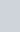6

Arrangement of Electron Pairs*

: :

Linear120°

A

Trigonal planar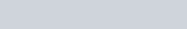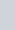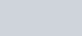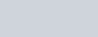120°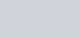Trigonal bipyramidal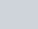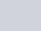Octahedral

# Molecular

Geometry”

B A B

LinearA

B- B

Trigonal planar

B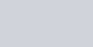B..„nA

Tetrahedral

B..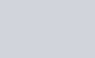“”A B

Trigonal bipyramidalB

Octahedral

0 ngñl +-J•fM hD&A f’otM say 0re• M tO O0I&cbon Or o

Table 10.2

Gaomatry of Simple b0oIecules end Rons in Whkh the Central Atom Haa Ona

Class of Total Number of Humber of Number of Arrangement of Geometry of Molecule Electron Pairs Bonding Pairs Lone Pairs Electron Pairs Molecule or Ion

ABLE 1 2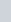ABLE 4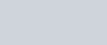Trignnal planar

B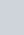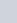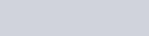Tetrahedral

Beni

Trigt›nul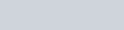AB_Ej 4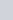ABLE

2 . ’B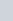Tctrahcdral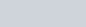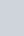B

B

4 ’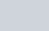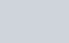B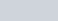Di lurteJ tetrahedron (of seosaw)

Tiigi›naJ hipyru›nictcI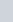AB 2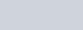2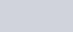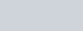6 4

B

Tiigonal bipyramidiil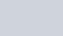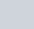B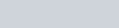B

Tiigonal bipyramidalB

OctafiedralOctaheiJraJ

T-shitped

Linear

fiquarc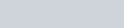Square planar